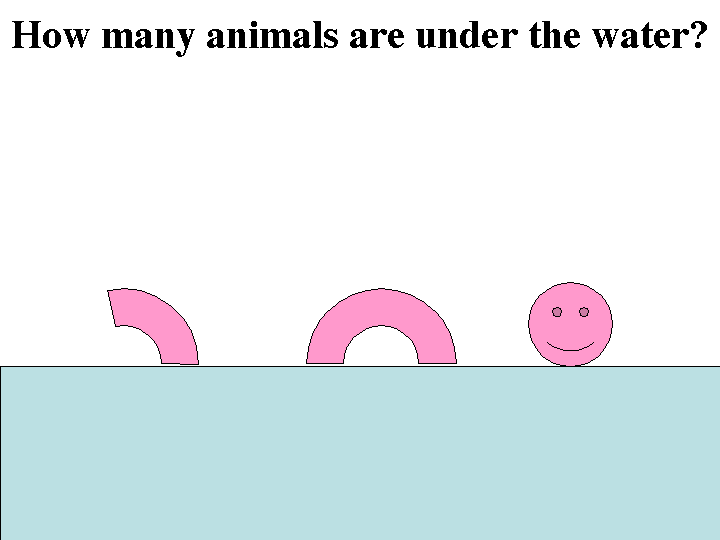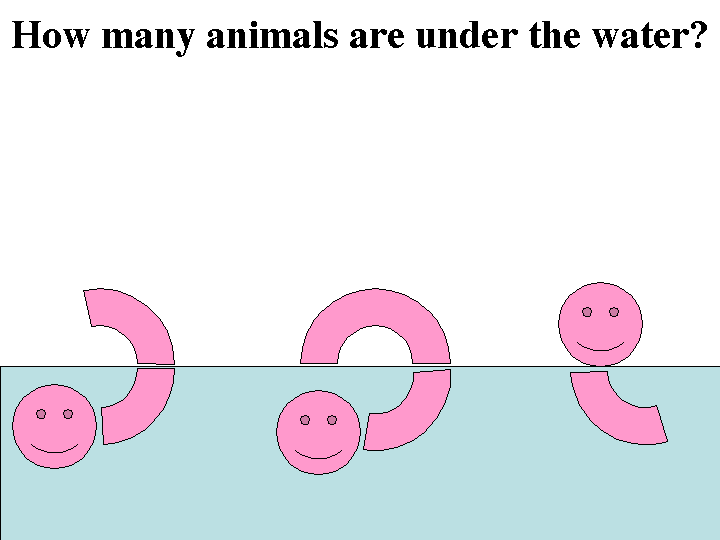devpsy.org  >  teaching  >  method  >  factor analysis k h g @ d e v p s y . o r g

Learn about factor analysis using a metaphorical sea monster only partially visible above the sea. The metaphor helps intuitively explain a statistical approach that is central in debates about the underlying nature of temperament (e.g., New York Longitudinal Study, Rothbart's IBQ), intelligence (e.g., "g"), personality (e.g., big 5), and attitudes (e.g., ABC model). Here are PowerPoint slides to teach factor analysis with metaphorical sea monsters.

# Factor Analysis

Factor analysis is a useful statistical method for finding the underlying structure of a pheonomeon. Without understanding factor analysis, it's hard to appreciate how developmental psychologists formed ideas about diverse topics such as temperament and intelligence. But how can we teach about it without using considerable class time explaining the mathematics more appropriate for a statistics or research methods class? In his introductory psychology textbook, Henry Gleitman introduced a metaphor of sea monsters and I expanded it into an animated-picture lecture component.

## From Particular Behaviors to Underlying Structure

How do developmental psychologists find underlying dimensions when we can only observe specific behaviors? I pose this question while making it concrete with the example of temperament (the first place my developmental students encounter factor analysis). How do we decide which behaviors cluster together into a temperament? How do we decide how many temperaments there are? Below is the figure I show for class, you could make a similar figure if intelligence is the place you first introduce factor analysis in your class.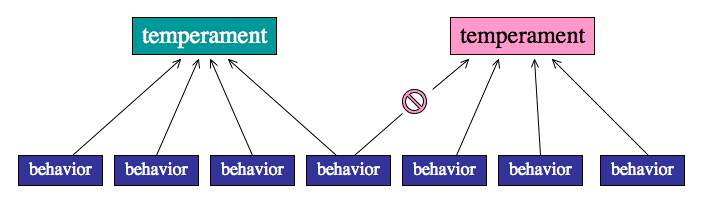## Observing Sea Monsters

Look at the picture below. How many sea monsters do you think there are. Note, if you do not see the figure moving, you may have set your web browser preferences to stop animated GIF files.Even though you can't see everything because the water obscures your view, you intuitively realize that there is probably only one sea monster because all of it's parts move together.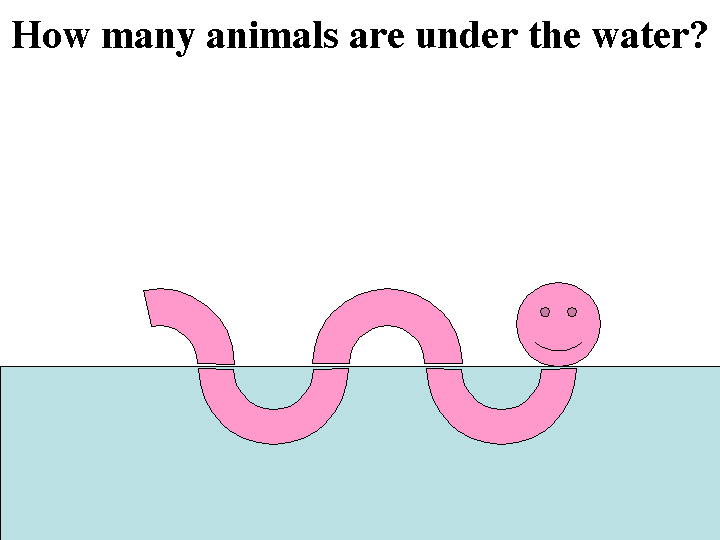Now let's try it again. How many sea monsters do you think there are?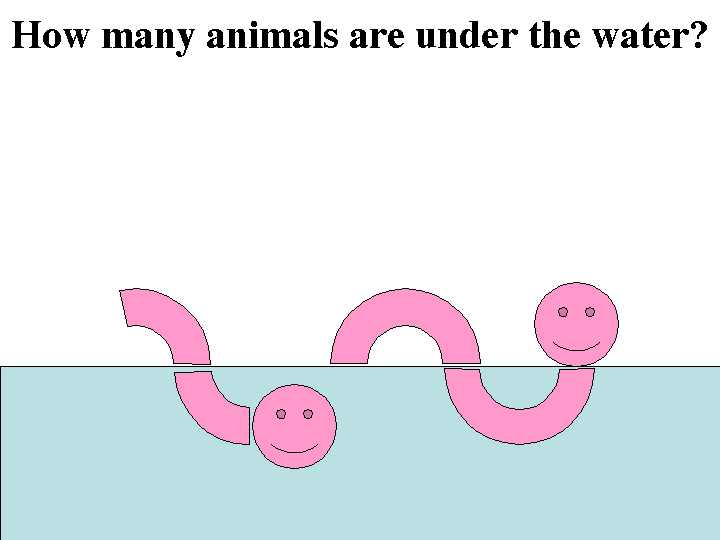Let's try one last time. How many sea monsters do you think there are?